Physics-
General
Easy

Question

# If the ratio of lengths, radii and Young’s modulus of steel and brass wires shown in the figure are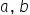and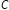, respectively. The ratio between the increase in lengths of brass and steel wires would be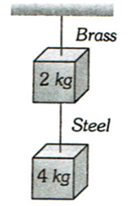## The correct answer is: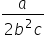### Given,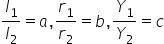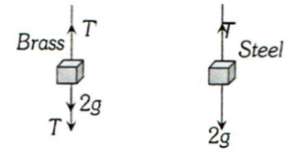Let Young’s modulus of steel be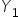, and that of brass be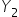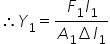(i)and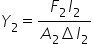(ii)Dividing Equation (i) by Equation (ii), we get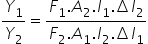(iii)Force on steel wire from free body diagram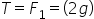NewtonForce on brass wire from free body diagram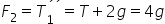NewtonNow, putting the value of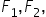in Equation (iii), we get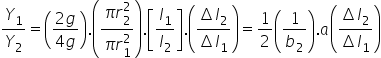#### With Turito Foundation.#### Get an Expert Advice From Turito.